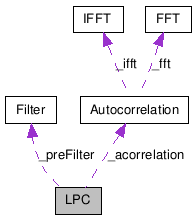# LPC Class Reference

Algorithm to calculate the Linear Predictive Coding of vectors of Real values. More...

`#include <LPC.h>`

Collaboration diagram for LPC:[legend]

List of all members.

## Public Member Functions

LPC (int inputSize=1024, int coefficientCount=15, Real preEmphasis=0.0)
~LPC ()
void process (const MatrixXR &frames, MatrixXR *lpcCoefficients, MatrixXR *reflectionCoefficients, MatrixXR *error)
int inputSize () const
void setInputSize (int size, bool callSetup=true)
int coefficientCount () const
void setCoefficientCount (int count, bool callSetup=true)
Real preEmphasis () const
void setPreEmphasis (Real coefficient, bool callSetup=true)

## Detailed Description

Algorithm to calculate the Linear Predictive Coding of vectors of Real values.

This class represents an object to perform a Linear Predictive Coding on vectors of Real values. Which is a useful technique for estimating a parametric representation of a spectrum magnitude. The algorithm estimates a set of M coefficients of a IIR filter whose frequency response approximates the vector of Reals passed as input.

This algorithm implements the Levinson-Durbin recursion for solving the following linear equation system:

R a = r

where R is the Toeplitz matrix made of the first M - 1 autocorrelation coefficients of the input vector and r is a vector made of M - 1 autocorrelation coefficients starting from the second of the input vector.

Optionally a pre-emphasis FIR filter may be applied to the input vector in order to enhance estimation of higher frequencies. The pre-emphasis filter consists of a 2 coefficient filter of the form b = [1, -b1] where usually:

0.96 <= b1 <= 0.99

The b1 coefficient defaults to 0, but can be specified using setPreEmphasis().

MelBands, Bands, MFCC

## Constructor & Destructor Documentation

 LPC ( int inputSize = `1024`, int coefficientCount = `15`, Real preEmphasis = `0.0` )

Constructs an LPC object with the specified inputSize, coefficientCount and preEmphasis settings.

Parameters:
 inputSize size of the inputs arrays, must be > 0. The algorithm performs faster for sizes which are a power of 2. coefficientCount number of coefficients to be estimated preEmphasis second coefficient of the FIR pre-emphasis filter

References setCoefficientCount(), setInputSize(), and setPreEmphasis().

 ~LPC ( )

Destroys the algorithm and frees its resources.

## Member Function Documentation

 void process ( const MatrixXR & frames, MatrixXR * lpcCoefficients, MatrixXR * reflectionCoefficients, MatrixXR * error )

Performs an LPC on each of the rows of frames. Puts the resulting LPC coefficients in the rows of lpcCoefficients, the reflection coefficients in reflectionCoefficients and the error in error.

Parameters:
 frames matrix of Real values. The number of columns of frames must be equal to the input size specified using setInputSize(). lpcCoefficients pointer to a matrix of Real values for the LPC coefficients. The matrix should have the same number of rows as frames and coefficientCount columns. reflectionCoefficients pointer to a matrix of Real values for the reflection coefficients. The matrix should have the same number of rows as frames and coefficientCount + 1 columns. error pointer to a matrix of Real values for the LPC error gain. The matrix should have the same number of rows as frames and 1 single column.
Note that if the output matrices are not of the required sizes they will be resized, reallocating a new memory space if necessary.

References Autocorrelation::process(), and Filter::process().

 int inputSize ( ) const

Returns the size of the input arrays. The default is 1024.

setInputSize()

 void setInputSize ( int size, bool callSetup = `true` )

Specifies the size of the input. The given size must be higher than 0. Note that if size is a power of 2 the algorithm will perform faster.

inputSize()

Referenced by LPC().

 int coefficientCount ( ) const

Returns the number of coefficients to be calculated. The default is 15.

setCoefficientCount()

 void setCoefficientCount ( int count, bool callSetup = `true` )

Specifies the count of coefficients to be calculated. The given count must be in the range between 0 and (input size - 1).

coefficientCount()

Referenced by LPC().

 Real preEmphasis ( ) const

Returns the second coefficient of the FIR preemphasis filter. The default is 0.0.

setPreEmphasis()

 void setPreEmphasis ( Real coefficient, bool callSetup = `true` )

Specifies the second coefficient of the FIR preemphasis filter.

Generated on Tue Mar 31 20:38:33 2009 for Loudia by1.5.6